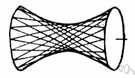# hyperboloid

(redirected from One-sheeted hyperboloid)
Also found in: Thesaurus, Encyclopedia.

## hy·per·bo·loid

(hī-pûr′bə-loid′)
n.
Either of two quadric surfaces generated by rotating a hyperbola about either of its main axes and having a finite center with certain plane sections that are hyperbolas and others that are ellipses or circles.

## hyperboloid

(haɪˈpɜːbəˌlɔɪd)
n
(Mathematics) a geometric surface consisting of one sheet, or of two sheets separated by a finite distance, whose sections parallel to the three coordinate planes are hyperbolas or ellipses. Equations x2/a2 + y2/b2z2/c2 = 1 (one sheet) or x2/a2y2/b2z2/c2 = 1 (two sheets) where a, b, and c are constants

## hy•per•bo•loid

(haɪˈpɜr bəˌlɔɪd)

n.
a quadric having a finite center and some of its plane sections hyperbolas. Equation: x2/a2 + y2/b2z2/c2= 1.
[1720–30]
 Noun 1hyperboloid - a quadric surface generated by rotating a hyperbola around its main axisquadric, quadric surface - a curve or surface whose equation (in Cartesian coordinates) is of the second degree